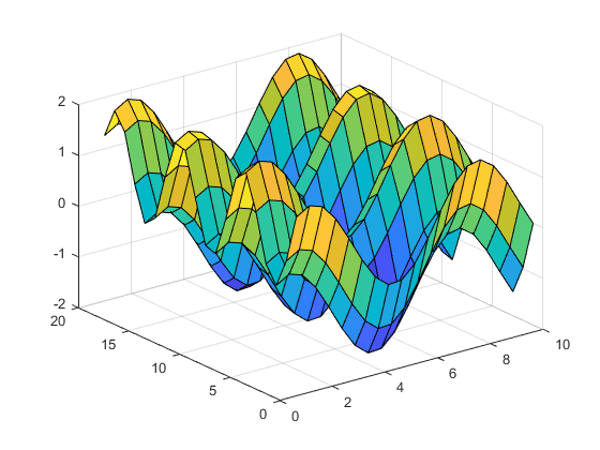Related Tags

surface plot
surface
plot
python plot

# How to create surface plots in PythonEducative Answers Team

## What are surface plots?

To visualize the relationship between three variables, we generally need a three-dimensional graph. Surface plots are two-dimensional​ diagrams of three-dimensional data. Surface plots show the functional relationship between independent and dependent variables.Example of a Surface Plot

In Python, we draw surface plots using matplotlib.

## Steps

1. Import libraries

We don’t need to import the entire matplotlib module, pyplot should be enough. Make sure to import ​numpy for any mathematics needed for the plot.

import numpy as np
import matplotlib.pyplot as plt
from mpl_toolkits.mplot3d import Axes3D


2. Introduce data

This usually comprises of two independent-variable arrays and a dependent variable array.

x = np.arange(0, 25, 1)
y = np.arange(0, 25, 1)
x, y = np.meshgrid(x, y)
z = x + y


3. Draw a plot

fig = plt.figure()
axes = fig.gca(projection ='3d')
axes.plot_surface(x, y, z)
plt.show()


## Code

import numpy as np # For mathematics, and making arrays
import matplotlib.pyplot as plt
from mpl_toolkits.mplot3d import Axes3D

# Arrays x, y and z for data plot visualization
x = np.arange(0, 25, 1)
y = np.arange(0, 25, 1)
# meshgrid makes a retangular grid out of two 1-D arrays.
x, y = np.meshgrid(x, y)
z = x**2 + y**2  # x^2+y^2

# surface plot for x^2 + y^2
fig = plt.figure() # creates space for a figure to be drawn

# Uses a 3d prjection as model is supposed to be 3D
axes = fig.gca(projection ='3d')

# Plots the three dimensional data consisting of x, y and z
axes.plot_surface(x, y, z)

# show command is used to visualize data plot
plt.show()


RELATED TAGS

surface plot
surface
plot
python plot